Concepts

Class 10
Chapter 10 Class 10 - Light - Reflection and Refraction

For a Convex Lens, object can be kept at different positions

Hence, we take different cases

## Case 1 - Object is Placed at infinity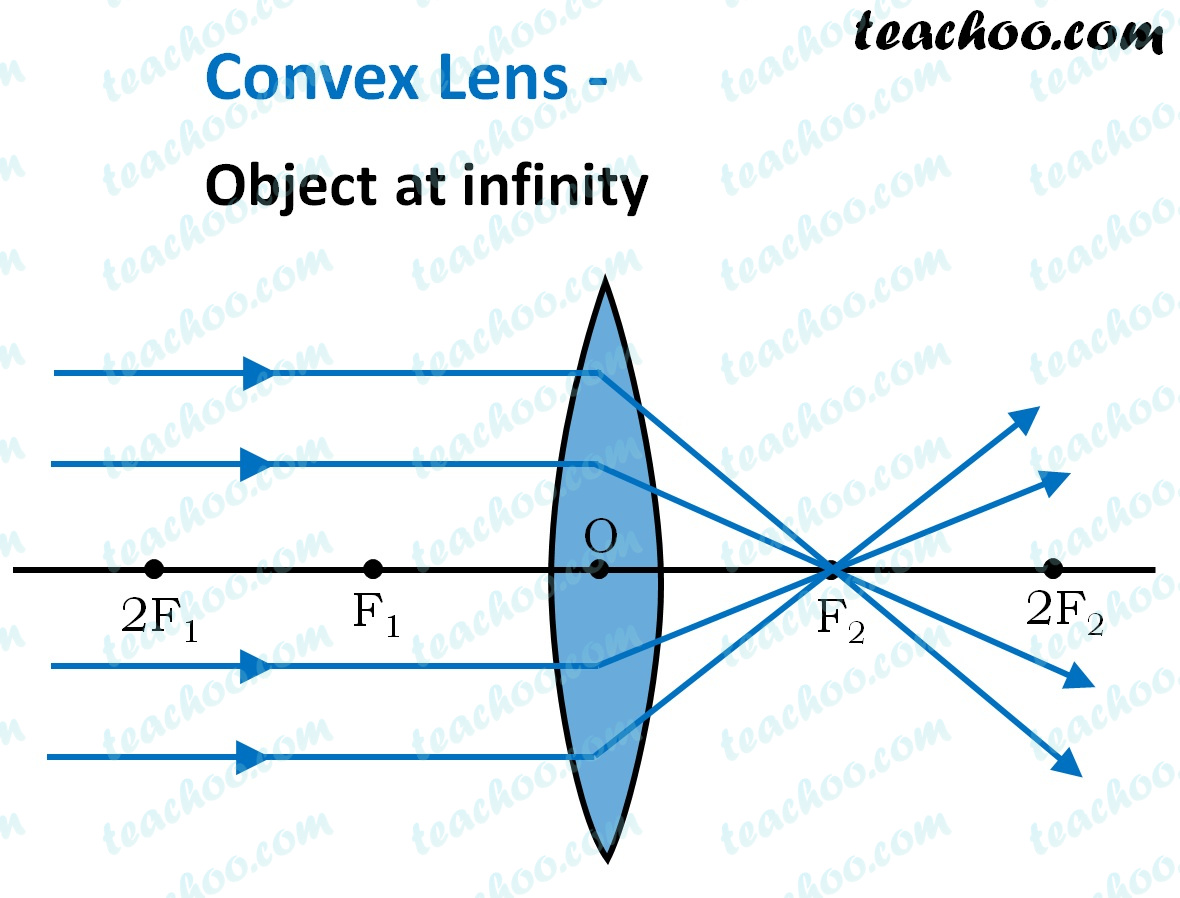In this Case, Object is kept far away from lens (almost at infinite distance)

So, we draw rays parallel to principal axis

Since ray parallel to principal axis passes through the Focus

Both rays meet at focus after refraction

Hence, Image is formed at F 2

And it is very very small

We can say that

• Image is Real
• Image is Inverted
• Image is Smaller than the Object (Highly Diminished)

## Case 2 - Object is beyond 2F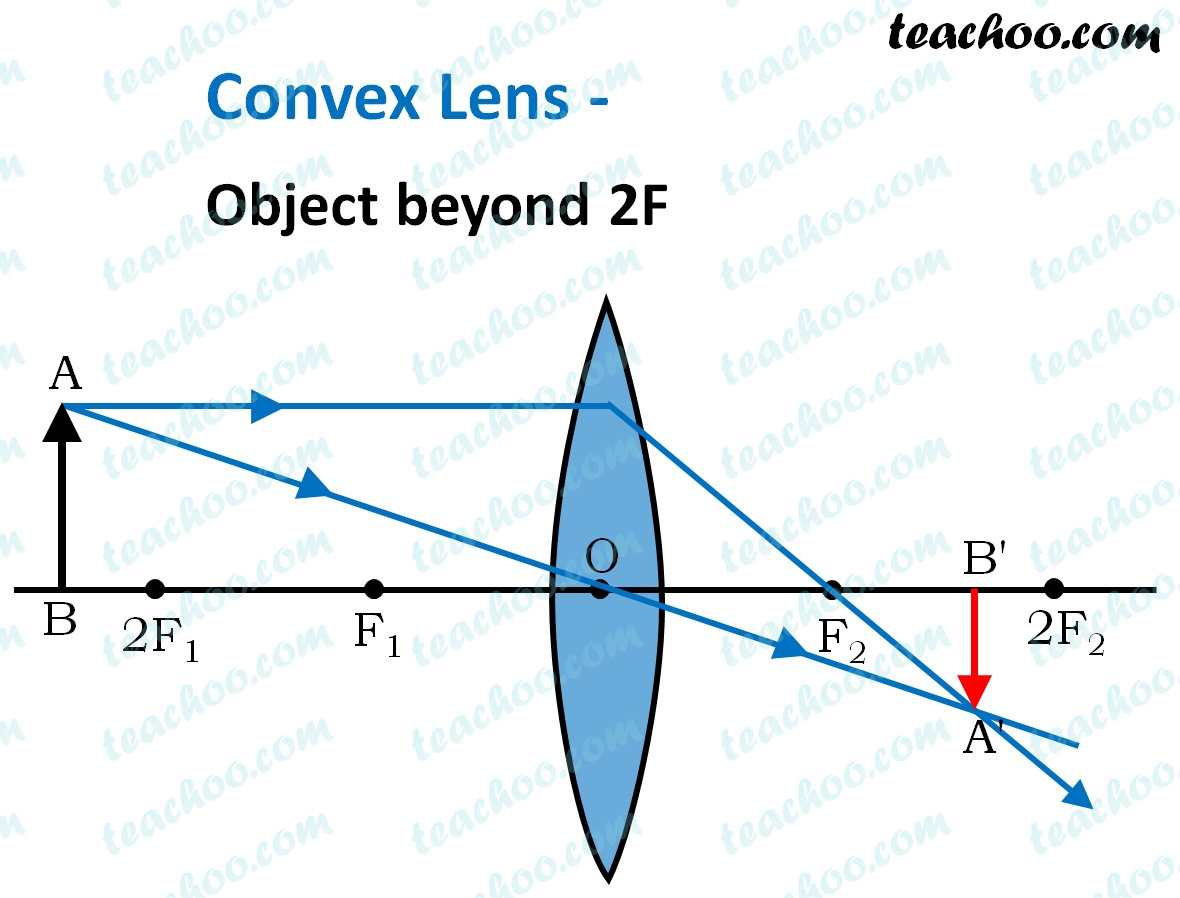Here, Object AB is beyond 2F 1

First, we draw a ray parallel to principal axis

So, it passes through focus after refraction

We draw another ray which passes through Optical Center

So, the ray will go through without any deviation

Where both rays meet is point A'

And the image formed is A'B'

This image is formed between F 2 and 2F 2

We can say that

• Image is Real
• Image is Inverted
• Image is Smaller than the Object (Diminished)

## Case 3 - Object is at 2F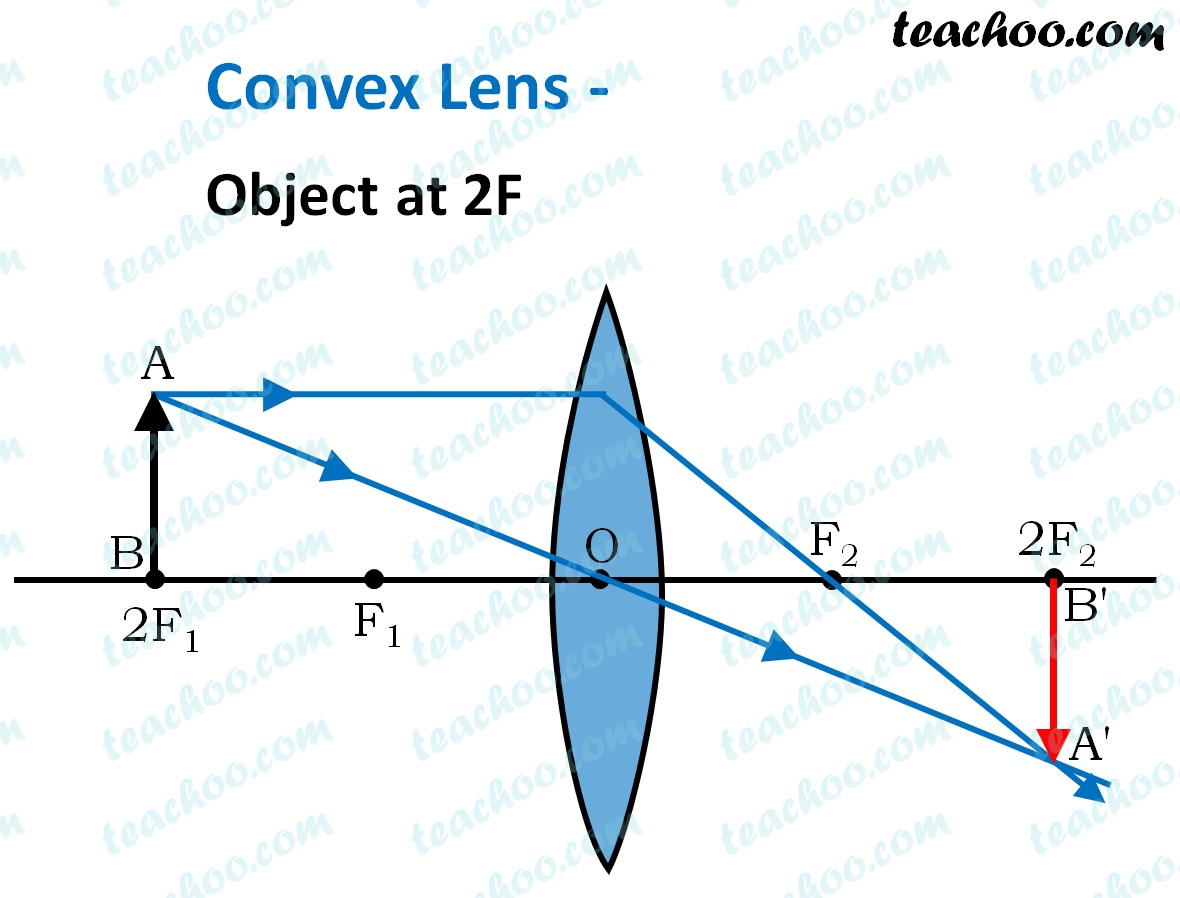Here, Object AB is at 2F 1

First, we draw a ray parallel to principal axis

So, it passes through focus after refraction

We draw another ray which passes through Optical Center

So, the ray will go through without any deviation

Where both refracted rays meet is point A'

And the image formed is A'B'

This image is formed at 2F 2

We can say that

• Image is Real
• Image is Inverted
• Image is exactly the same size as that of Object

## Case 4 - Object is between F and 2F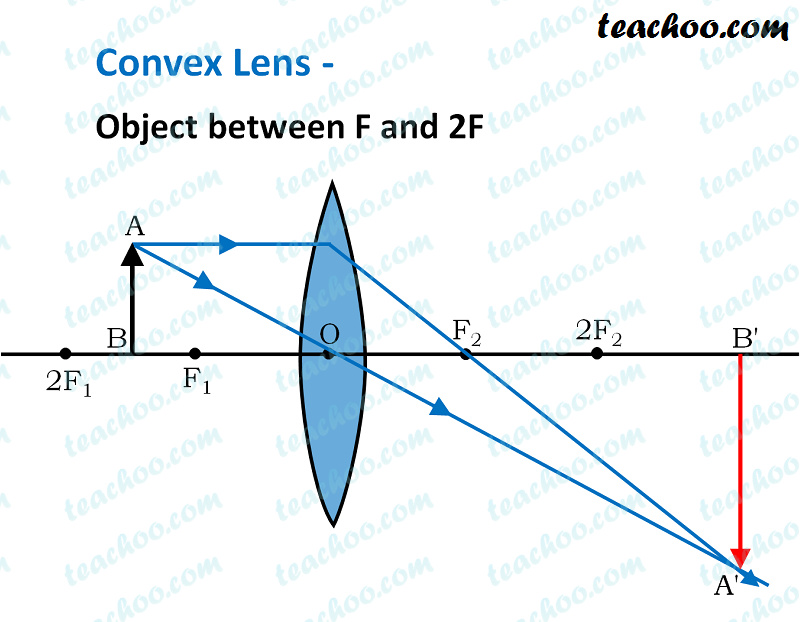Here, Object AB is kept between F 1 and 2F 1

First, we draw a ray parallel to principal axis

So, it passes through focus after refraction

We draw another ray which passes through Optical Center

So, the ray will go through without any deviation

Where both refracted rays meet is point A'

And the image formed is A'B'

This image is formed between beyond 2F 2

We can say that

• Image is Real
• Image is Inverted
• Image is larger than the object (Magnified)

## Case 5 - Object is at F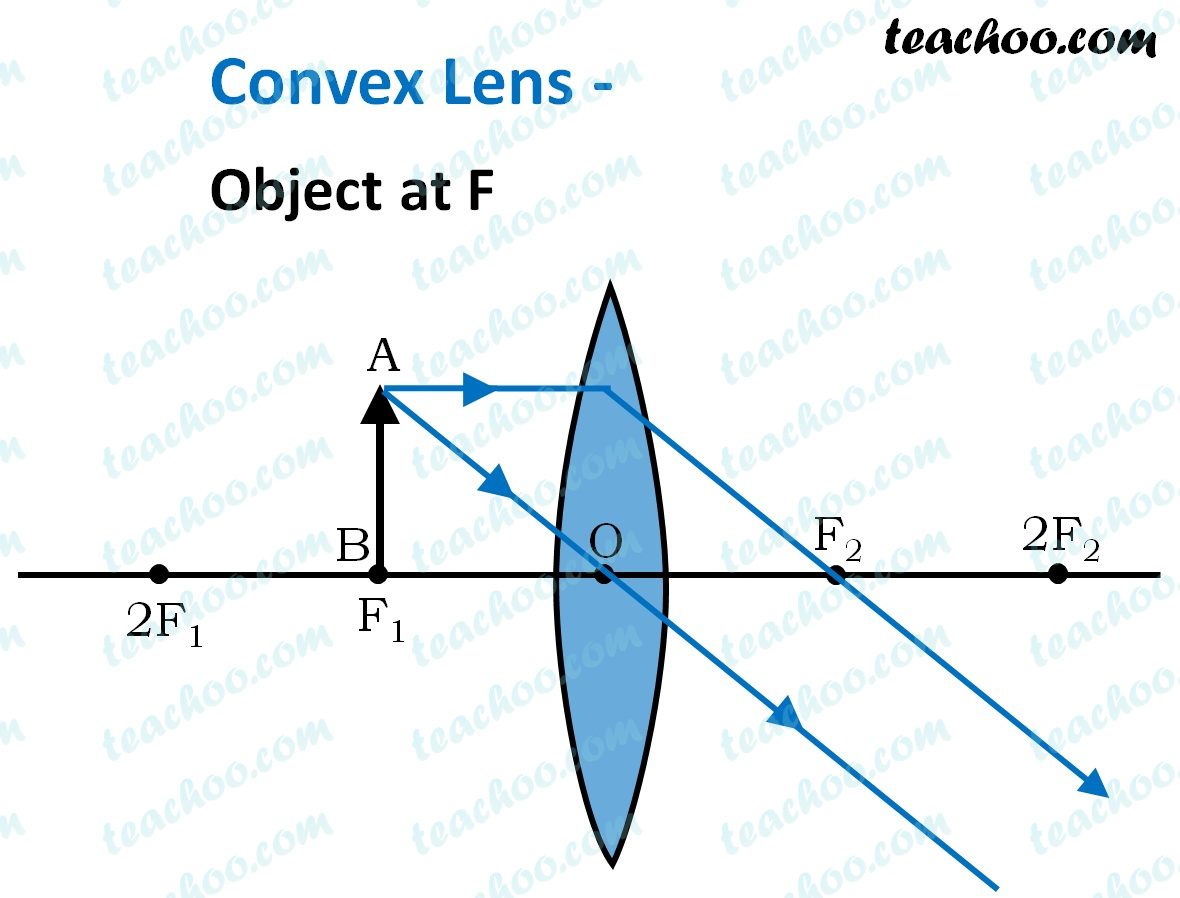Here, Object AB is kept at F 1

First, we draw a ray parallel to principal axis

So, it passes through focus after refraction

We draw another ray which passes through Optical Center

So, the ray will go through without any deviation

We observe that both rays are parallel to each other

Hence we can say that they meet at infinity.

Hence, the rays form an image at infinity

And image formed would be larger than the object

We can say that

• Image is Real
• Image is Inverted
• Image is larger than the object (Magnified)

## Case 6 - Object is between O and F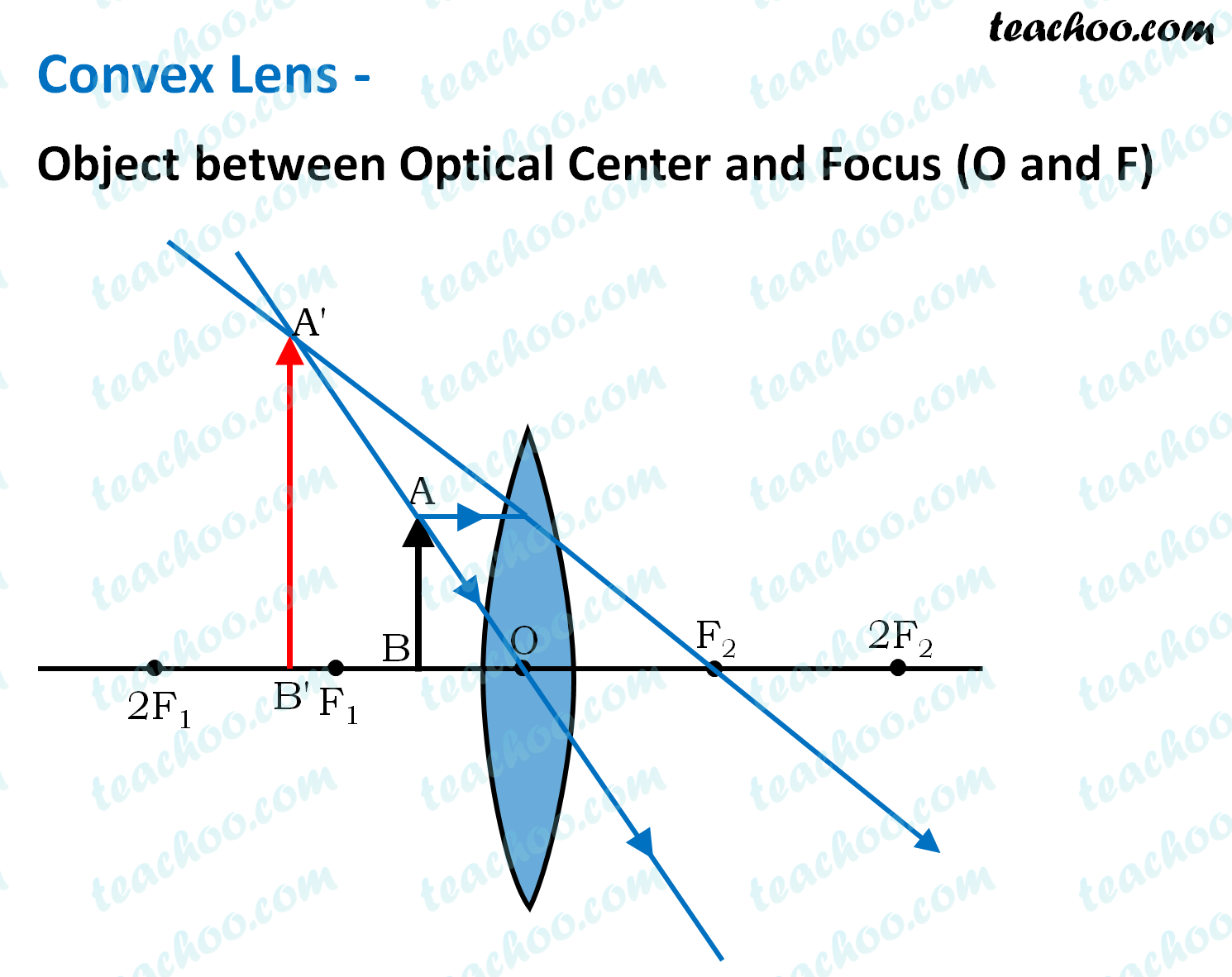Here, Object AB is kept between O and F 1

First, we draw a ray parallel to principal axis

So, it passes through focus after refraction

We draw another ray which passes through Optical Center

So, the ray will go through without any deviation

We observe that both refracted rays are diverging

It means that they would have met at some point

Hence, we extend both rays behind the lens

We see that the rays form an image behind the lens (on the left side).

So, the image is virtual

And image formed would be larger than the object

We can say that

• Image is virtual (behind the lens)
• Image is erect
• Image is larger than the object (Magnified)

## To summarise

 Position of the object Position of the image Relative size of the image Nature of the image At infinity At focus F 2 Highly diminished,  point-sized Real and inverted Beyond 2F 1 Between F 2 and 2F 2 Diminished Real and inverted At 2F 1 At 2F 2 Same Size Real and inverted Between F 1 and 2F 1 Beyond 2F 2 Enlarged Real and inverted At focus F 1 At infinity Infinitely large or highly enlarged Real and inverted Between focus F 1 and optical Center O On the same side of the lens as the object Enlarged Virtual  and erect

Learn in your speed, with individual attention - Teachoo Maths 1-on-1 Class